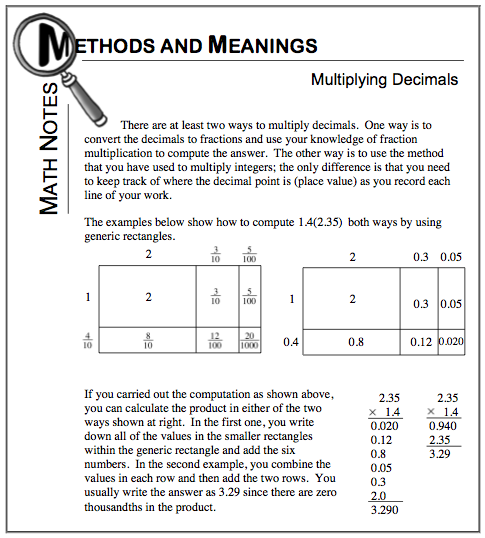### Home > CC1 > Chapter 6 > Lesson 6.2.4 > Problem6-110

6-110.

Evaluate the expressions below for the given values of the variables. Homework Help ✎

For the expression below, you can substitute the values given for each variable and then simplify the expression.
It is important to remember that $2p$ really means two p's or $(2) \times (p)$.
$2p + 8 \text{ for } p = 4$
$2(4) + 8 = 8 + 8 = 16$
And, remember to follow the Order of Operations!

1. $6j−3\ \text{for}\ j=4$

Be sure to show all of your work!
This way you will be less likely to make mistakes.

$21$

2. $b(b)+5 \text{ for } b=3$

If the variable is substituted here, the expression becomes $3(3) + 5$.
Can you simplify it now?

$14$

3. $8+4k \text{ for } k=3.5$

• Remember to follow the rules of multiplication. Refer to the Math Box below for help with multiplying decimals.